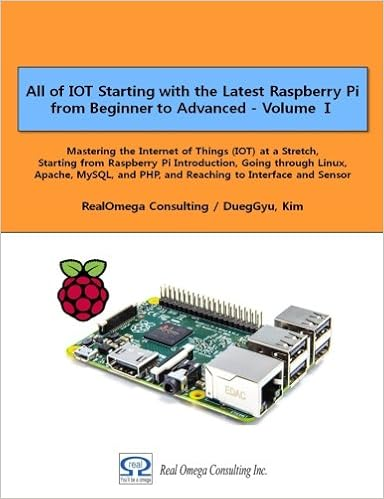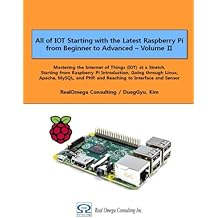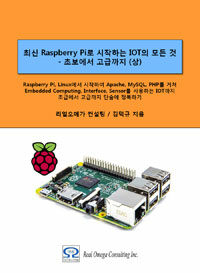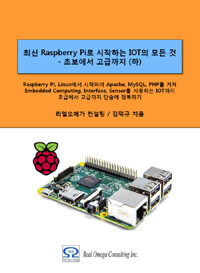# Raspberry Pi_Eng_23.2.1 Features of Mathematica or Wolfram language

#### Published Book on AmazonAll of IOT Starting with the Latest Raspberry Pi from Beginner to Advanced – Volume 1All of IOT Starting with the Latest Raspberry Pi from Beginner to Advanced – Volume 2

#### 출판된 한글판 도서최신 라즈베리파이(Raspberry Pi)로 시작하는 사물인터넷(IOT)의 모든 것 – 초보에서 고급까지 (상)최신 라즈베리파이(Raspberry Pi)로 시작하는 사물인터넷(IOT)의 모든 것 – 초보에서 고급까지 (하)

## 23.2Mathematica or Wolfram language

### 23.2.1Features

Mathematica is a programming tool specialized for numerical analysis that facilitates the professional mathematical calculations needed in science, mathematics, computers, and engineering. It was developed in 1988 by Wolfram Research.

The developer, Stephen Wolfram is originally a particle physicist. While studying particle physics, he created a program for complex mathematical calculations and digged into program development. After all, he abandoned physics and devoted himself to development of the program. Mathematica is the program he developed in this way.

Mathematica is a popular tool for complex and specialized mathematical calculations, such as differential and integral calculations, and can do huge calculations quickly.

Mathematica is similar in use to tools like Maple and MATLAB, but the basic approach is different. These tools solve the problem generally by using numerical analysis method when doing mathematical calculations. Also, for numerical computation itself, it can be much faster to use tools like Fortran or C. But the basic feature of Mathematica is that it can do symbolic mathematics. Mathematica solves problems in analytical ways such as solving equation or deriving relational expression, by organizing complex mathematical expressions and mathematically producing optimal results.

It was available free of charge on Raspberry Pi since 2013, and is available as basic software in Raspbian. When you install Raspbian on Raspberry Pi, you can see that Mathematica and Wolfram are installed on the desktop.

wolfram                 -- http://www.raspberrypi.org/tag/wolfram/

mathematica                       -- http://www.raspberrypi.org/tag/mathematica/

wolfram language     -- http://www.raspberrypi.org/tag/wolfram-language/

wolfram research      -- http://www.raspberrypi.org/tag/wolfram-research/